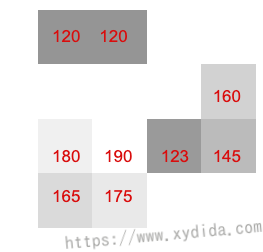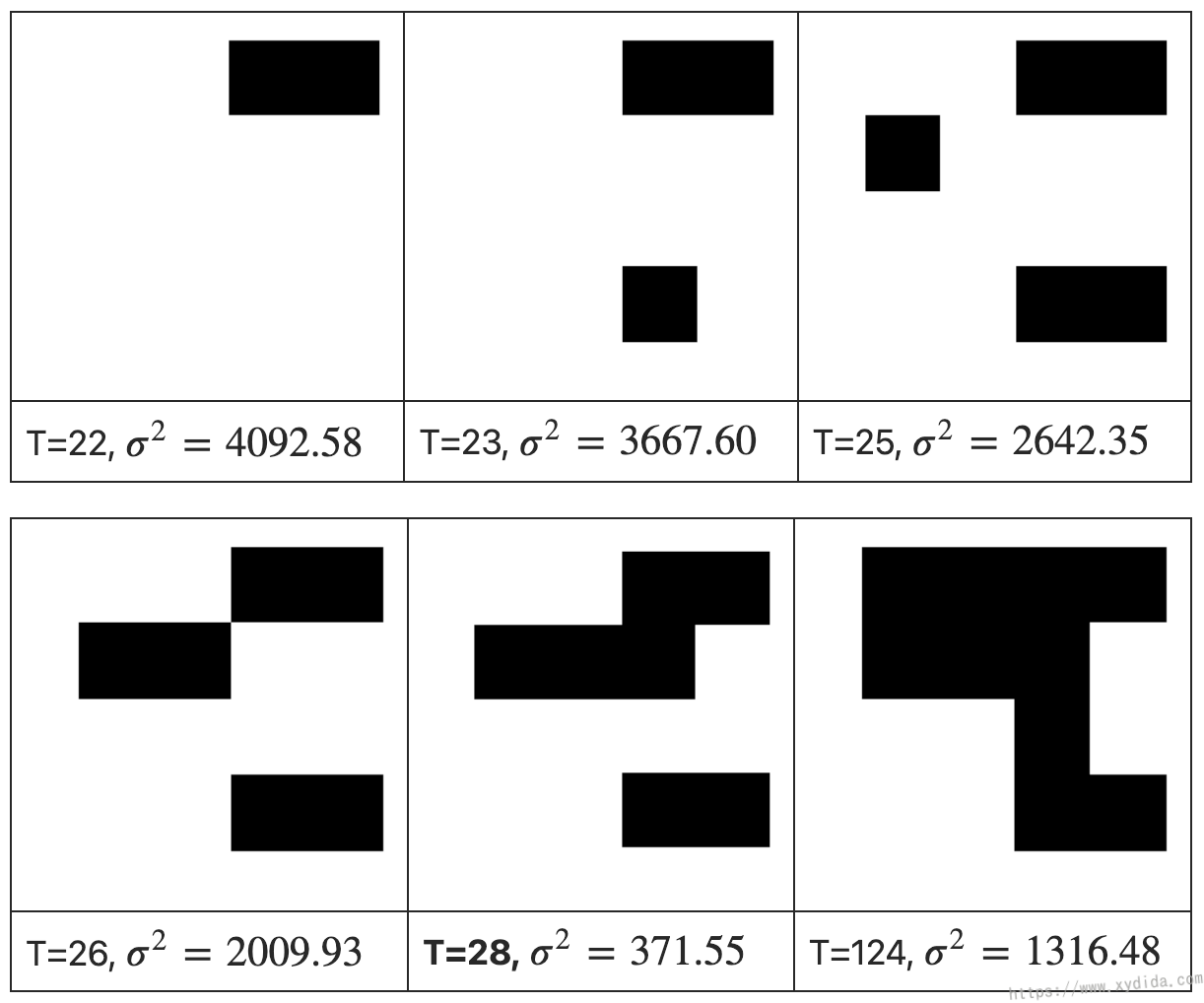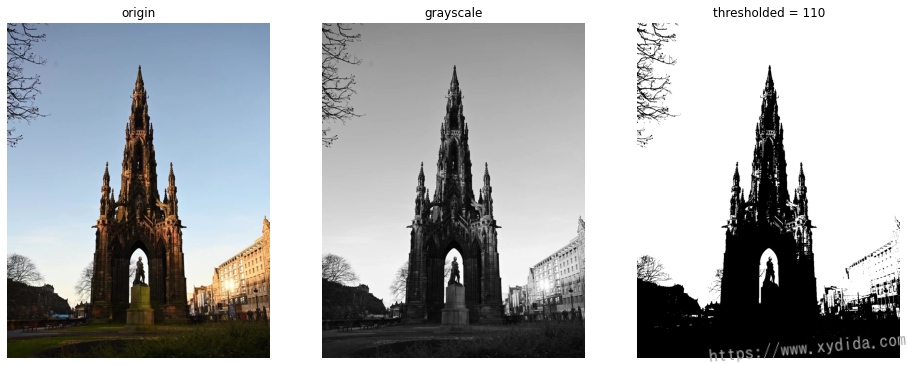# Image thresholding with Otsu method

Table of content

Image thresholding is a process for separating the foreground and background of the image. There are lots of methods for image thresholding, Otsu method is one of the methods proposed by Nobuyuki Otsu. The Otsu algorithm is a variance-based way to automatically find a threshold value by which the weighted variance between foreground and background is the least.

With different threshold value, the pixel values of foreground and background are various. Hence, both pixels have different variance for different thresholding. The key of Otsu algorithm is to calculate the total variance from the two variances of both distributions. The process needs to iterate through all the possible threshold vlaues and find a threshold that makes the total variance is smallest.

# Algorithm

The Otsu method iteratively search for the threshold value that minimizes the weighted sum of variance of the two classes (foreground and background). The pixel value is usually betwwen 0-255, So if the threshold value is 100, then all the pixels with values that less than 100 will be the background and all the pixels with values that greater than or equal to 100 will be the foreground of the image. The formula for find the variance at any threshold of t is given by:

$\theta^2(t) = \omega_{bg}(t)\theta^2_{bg}(t) + \omega_{fg}(t)\theta^2_{fg}(t) \tag{1}$

where $\omega_{bg}$ and $\omega_{fg}$ are the probability of the number of pixels for each class at threshold t and $\theta^2$ represents the variance of color values.

So, what is the probability in eq. 1? Here is a detail explain, let’s assume:

$P_{all}$ be the total count of pixels in an image,
$P_{bg}(t)$ be the count of background pixels at threshold t,
$P_{fg}(t)$ be the count of foreground pixels at threshold t

So, the weights are given by:

$\omega_{bg}(t) = \frac{P_{bg}(t)}{P_{all}} \\ \omega_{fg}(t) = \frac{P_{fg}(t)}{P_{all}} \tag{2}$

The variance can be calculated using the below formula:

$\theta^2(t) = \frac{\sum(x_{i}-\bar{x})^2}{N} \tag{3}$

where,

$x_{i}$ is the value of pixel at i in the group (bg or fg)
$\bar{x}$ is the mean of pixel values in the group (bg or fg)
$N$ is the number of pixels.

Now, I’ll give an example to understand the algorithm. here is an image with 4x4 size, the Threshold T is 100:The foreground is the pixel value which greater than or equal to 100, the background is the pixel value less than 100:Now the pixel values have been separated to two parts. Thus I can get:

$P_{all} = 4*4 = 16$
$P_{bg} = 7$
$P_{fg} = 9$

The weights can be:

$\omega_{bg}(t=100) = \frac{P_{bg}}{P_{all}} = \frac{7}{16} = 0.44$
$\omega_{fg}(t=100) = \frac{P_{fg}}{P_{all}} = \frac{9}{16} = 0.56$

The means of foreground and background:

$\bar{x}_{bg} = \frac{21+22+25+26+27+23+24}{7} = 24$
$\bar{x}_{fg} = \frac{120+120+160+180+190+123+145+165+175}{9} = 153.1$

The variances:

$\theta^2_{bg}(t=100) = \frac{(21-24)^2 + (22-24)^2 + ... + (24-24)^2}{7} = 4$
$\theta^2_{fg}(t=100) = \frac{(120-153.1)^2 + (120-153.1)^2 + ... + (175-153.1)^2}{9} = 657.43$

Take all the values into the Eq. (1):

$\theta^2(t=100) = 0.44*4 + 0.56*657.43 = 369.9208$

Also, we can find other values on different threshould t.From the above experiments, the least variance can be achieved at a threshold of 28.

# Code

Output:Reference: